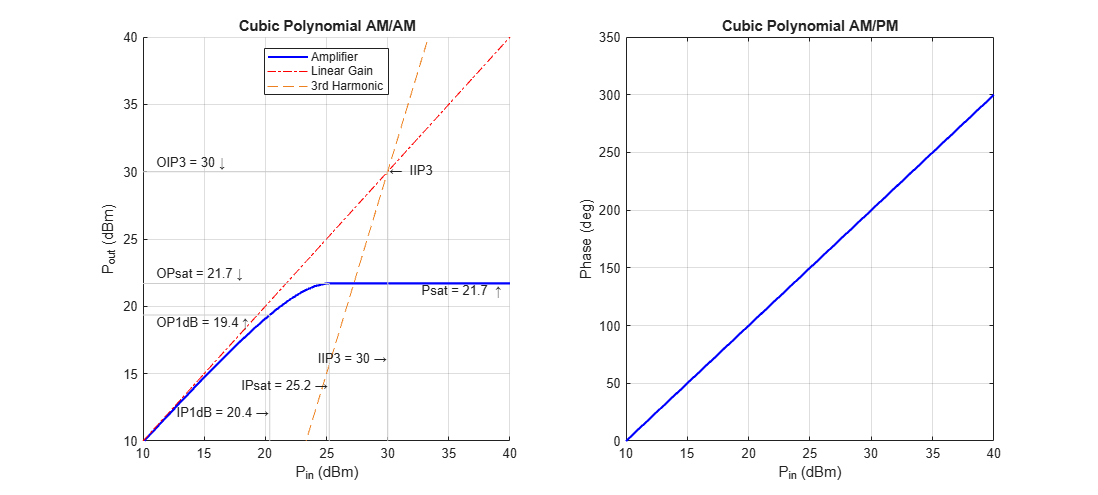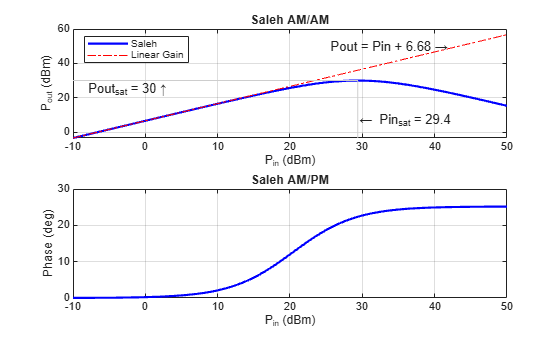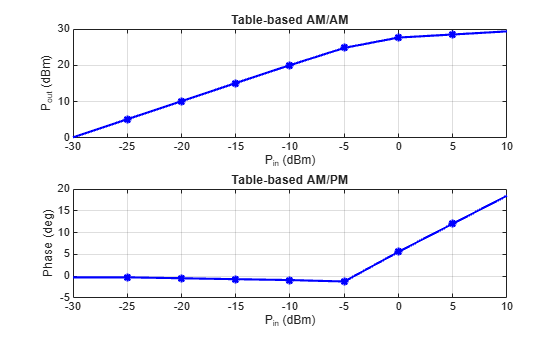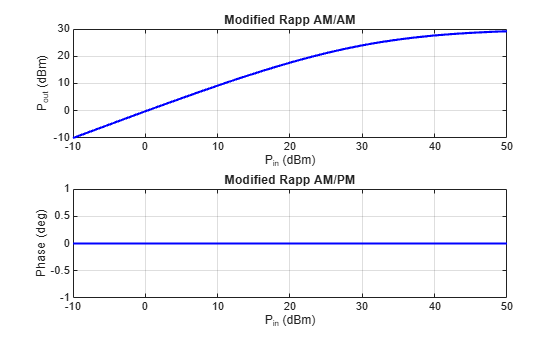# plot

Plot nonlinearity AM/AM and AM/PM characteristics

## Syntax

``plot(mnl)``
``plot(mnl,'Gain')``
``mnlplot = plot(___)``

## Description

example

````plot(mnl)` plots the output signal power and the phase change versus the input signal power. This syntax is equivalent to `plot(mnl,'Pout')`.```
````plot(mnl,'Gain')` plots the gain and the phase change versus the input signal power.```
````mnlplot = plot(___)` returns a handle to the figure containing the generated plot. Specify an input argument combination from any of the previous syntaxes.```

## Examples

collapse all

Plot the amplifier output power and phase response for various model methods.

Create a memoryless nonlinearity impairment System object for each of the nonlinearity modeling methods.

```ampCubicPoly= comm.MemorylessNonlinearity('Method','Cubic polynomial'); ampHyperbolic = comm.MemorylessNonlinearity('Method','Hyperbolic tangent'); ampGhorbani = comm.MemorylessNonlinearity('Method','Ghorbani model'); ampSaleh = comm.MemorylessNonlinearity('Method','Saleh model'); ampModRapp = comm.MemorylessNonlinearity('Method','Modified Rapp model'); ampLookupTable = comm.MemorylessNonlinearity('Method','Lookup table','ReferenceImpedance',50);```

Use the `plot` object function of the `comm.MemorylessNonlinearity` System object to show the response curves for the output power and phase for the each of the amplifier models.

`plot(ampCubicPoly);``plot(ampHyperbolic);``plot(ampSaleh);``plot(ampLookupTable);``plot(ampGhorbani);``plot(ampModRapp);`## Input Arguments

collapse all

Memoryless nonlinearity, specified as a `comm.MemorylessNonlinearity` System object.

## Output Arguments

collapse all

Plot figure, returned as a `Figure` object created using the `figure` function. Use the `Figure` object to query or modify properties of the figure after it is created.